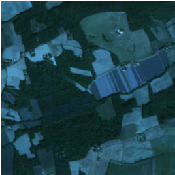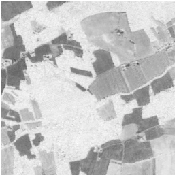Chapter 17Image Simulation

This chapter deals with image simulation algorithm. Using objects transmittance and reflectance and sensor characteristics, it can be possible to generate realistic hyperspectral synthetic set of data. This chapter includes PROSPECT (leaf optical properties) and SAIL (canopy bidirectional reflectance) model. Vegetation optical properties are modeled using PROSPECT model .

17.1 PROSAIL model

PROSAIL  model is the combinaison of PROSPECT leaf optical properties model and SAIL canopy bidirectional reflectance model. PROSAIL has also been used to develop new methods for retrieval of vegetation biophysical properties. It links the spectral variation of canopy reflectance, which is mainly related to leaf biochemical contents, with its directional variation, which is primarily related to canopy architecture and soil/vegetation contrast. This link is key to simultaneous estimation of canopy biophysical/structural variables for applications in agriculture, plant physiology, or ecology, at different scales. PROSAIL has become one of the most popular radiative transfer tools due to its ease of use, general robustness, and consistent validation by lab/field/space experiments over the years. Here we present a first example, which returns Hemispheric and Viewing reflectance for wavelength sampled from 400 to 2500nm. Inputs are leaf and Sensor (intrinsic and extrinsic) characteristics.

The source code for this example can be found in the file
Examples/Simulation/ProsailModel.cxx.

This example presents how to use PROSAIL (Prospect + Sail) model to generate viewing reflectance from leaf parameters, vegetation, and viewing parameters. Output can be used to simulate image for example.

Let’s look at the minimal code required to use this algorithm. First, the following headers must be included.

#include "otbSailModel.h"
#include "otbProspectModel.h"

We now define leaf parameters, which characterize vegetation composition.

typedef otb::LeafParameters LeafParametersType;

Next the parameters variable is created by invoking the New() method and assigning the result to a itk::SmartPointer .

LeafParametersType::Pointer leafParams = LeafParametersType::New();

Leaf characteristics is then set. Input parameters are :

• Chlorophyll concentration (Cab) in μg∕cm2.
• Carotenoid concentration (Car) in μg∕cm2.
• Brown pigment content (CBrown) in arbitrary unit.
• Water thickness EWT (Cw) in cm.
• Dry matter content LMA (Cm) in g∕cm2.
• Leaf structure parameter (N).

double Cab = static_cast<double> (atof(argv));
double Car = static_cast<double> (atof(argv));
double CBrown = static_cast<double> (atof(argv));
double Cw = static_cast<double> (atof(argv));
double Cm = static_cast<double> (atof(argv));
double N = static_cast<double> (atof(argv));

leafParams->SetCab(Cab);
leafParams->SetCar(Car);
leafParams->SetCBrown(CBrown);
leafParams->SetCw(Cw);
leafParams->SetCm(Cm);
leafParams->SetN(N);

Leaf parameters are used as prospect input

typedef otb::ProspectModel ProspectType;
ProspectType::Pointer prospect = ProspectType::New();

prospect->SetInput(leafParams);

Now we use SAIL model to generate transmitance and reflectance spectrum. SAIL model is created by invoking the New() method and assigning the result to a itk::SmartPointer .

sail input parameters are :

• leaf area index (LAI).
• average leaf angle (Angle) in deg.
• soil coefficient (PSoil).
• hot spot (HSpot).
• solar zenith angle (TTS) in deg.
• observer zenith angle (TTO) in deg.
• azimuth (PSI) in deg.

double LAI = static_cast<double> (atof(argv));
double Angle = static_cast<double> (atof(argv));
double PSoil = static_cast<double> (atof(argv));
double Skyl = static_cast<double> (atof(argv));
double HSpot = static_cast<double> (atof(argv));
double TTS = static_cast<double> (atof(argv));
double TTO = static_cast<double> (atof(argv));
double PSI = static_cast<double> (atof(argv));

typedef otb::SailModel SailType;

SailType::Pointer sail = SailType::New();

sail->SetLAI(LAI);
sail->SetAngl(Angle);
sail->SetPSoil(PSoil);
sail->SetSkyl(Skyl);
sail->SetHSpot(HSpot);
sail->SetTTS(TTS);
sail->SetTTO(TTO);
sail->SetPSI(PSI);

Reflectance and Transmittance are set with prospect output.

sail->SetReflectance(prospect->GetReflectance());
sail->SetTransmittance(prospect->GetTransmittance());

The invocation of the Update() method triggers the execution of the pipeline.

sail->Update();

GetViewingReflectance method provides viewing reflectance vector (size Nx2, where N is the number of sampled wavelength values, columns corresponds respectively to wavelength and viewing reflectance) by calling GetResponse. GetHemisphericalReflectance method provides hemispherical reflectance vector (size Nx2, where N is the number ofsampled wavelength values, columns corresponds to wavelength and hemispherical reflectance) by calling GetResponse.

Note that PROSAIL simulation are done for 2100 samples starting from 400nm up to 2500nm

for (unsigned int i = 0; i < sail->GetViewingReflectance()->Size(); ++i)
{
std::cout << "wavelength  : ";
std::cout << sail->GetViewingReflectance()->GetResponse()[i].first;
std::cout << ". Viewing reflectance ";
std::cout << sail->GetViewingReflectance()->GetResponse()[i].second;
std::cout << ". Hemispherical reflectance ";
std::cout << sail->GetHemisphericalReflectance()->GetResponse()[i].second;
std::cout << std::endl;
}

here you can found example parameters :

• Cab 30.0
• Car 10.0
• CBrown 0.0
• Cw 0.015
• Cm 0.009
• N 1.2
• LAI 2
• Angle 50
• PSoil 1
• Skyl 70
• HSpot 0.2
• TTS 30
• TTO 0
• PSI 0

17.2 Image Simulation

Here we present a complete pipeline to simulate image using sensor characteristics and objects reflectance and transmittance properties. This example use :

• input image
• label image : describes image object properties.
• label properties : describes each label characteristics.
• acquisition rarameter file : file containing the parameters for the acquisition.
• RSR File : File name for the relative spectral response to be used.
• sensor FTM file : File name for sensor spatial interpolation.

Algorithm is divided in following step :

1. LAI (Leaf Area Index) image estimation using NDVI formula.
2. Sensor Reduce Spectral Response (RSR) using PROSAIL reflectance output interpolated at sensor spectral bands.
3. Simulated image using Sensor RSR and Sensor FTM.

17.2.1 LAI image estimation

The source code for this example can be found in the file
Examples/Simulation/LAIFromNDVIImageTransform.cxx.

This example presents a way to generate LAI (Leaf Area Index) image using formula dedicated to Formosat2. LAI Image is used as an input in Image Simulation process.

Let’s look at the minimal code required to use this algorithm. First, the following headers must be included.

#include "otbMultiChannelRAndNIRIndexImageFilter.h"

Filter type is a generic otb::MultiChannelRAndNIRIndexImageFilter using Formosat2 specific LAI otb::LAIFromNDVIFormosat2Functor .

typedef otb::Functor::LAIFromNDVIFormosat2Functor
<InputImageType::InternalPixelType,
InputImageType::InternalPixelType, OutputImageType::PixelType> FunctorType;
typedef otb::MultiChannelRAndNIRIndexImageFilter
<InputImageType, OutputImageType, FunctorType>
MultiChannelRAndNIRIndexImageFilterType;

Next the filter is created by invoking the New() method and assigning the result to a itk::SmartPointer .

MultiChannelRAndNIRIndexImageFilterType::Pointer filter
= MultiChannelRAndNIRIndexImageFilterType::New();

filter input is set with input image

then red and nir channels index are set using SetRedIndex() and SetNIRIndex()

unsigned int redChannel = static_cast<unsigned int> (atoi(argv));
unsigned int nirChannel = static_cast<unsigned int> (atoi(argv));
filter->SetRedIndex(redChannel);
filter->SetNIRIndex(nirChannel);

The invocation of the Update() method triggers the execution of the pipeline.

filter->Update();Figure 17.1: LAI generation (right) from NDVI applied on Formosat 2 Image (left) .

Figure 17.1 illustrates the LAI generation using Formosat 2 data.

17.2.2 Sensor RSR Image Simulation

The source code for this example can be found in the file
Examples/Simulation/LAIAndPROSAILToSensorResponse.cxx.

The following code is an example of Sensor spectral response image generated using image of labeled objects image, objects properties (vegetation classes are handled using PROSAIL model, non-vegetation classes are characterized using Aster database characteristics provided by a text file), acquisition parameters, sensor characteristics, and LAI (Leaf Area Index) image.

Sensor RSR is modeled by 6S (Second Simulation of a Satellite Signal in the Solar Spectrum) model . Detailed information about 6S can be found here.

Let’s look at the minimal code required to use this algorithm. First, the following headers must be included.

#include "otbLeafParameters.h"
#include "otbReduceSpectralResponse.h"
#include "otbImageSimulationMethod.h"
#include "otbSpatialisationFilter.h"
#include "otbAttributesMapLabelObject.h"
#include "itkTernaryFunctorImageFilter.h"
#include "otbRAndNIRIndexImageFilter.h"
#include "otbVectorDataToLabelMapWithAttributesFilter.h"

ImageUniqueValuesCalculator class is defined here. Method GetUniqueValues() returns an array with all values contained in an image. This class is implemented and used to test if all labels in labeled image are present in label parameter file.

template < class TImage >
class ITK_EXPORT ImageUniqueValuesCalculator : public itk::Object
{
public:
typedef ImageUniqueValuesCalculator<TImage>   Self;
typedef itk::Object                           Superclass;
typedef itk::SmartPointer<Self>               Pointer;
typedef itk::SmartPointer<const Self>         ConstPointer;

itkNewMacro(Self);

itkTypeMacro(ImageUniqueValuesCalculator, itk::Object);

itkStaticConstMacro(ImageDimension, unsigned int,
TImage::ImageDimension);

typedef typename TImage::PixelType PixelType;

typedef TImage ImageType;

typedef std::vector<PixelType>           ArrayType;

typedef typename ImageType::Pointer      ImagePointer;
typedef typename ImageType::ConstPointer ImageConstPointer;

virtual void SetImage( const ImageType  image )
{
if ( m_Image != image )
{
m_Image = image;
this->Modified();
}
}

ArrayType GetUniqueValues() const
{
ArrayType uniqueValues;
if( !m_Image )
{
return uniqueValues;
}

itk::ImageRegionConstIterator< ImageType > it( m_Image,
m_Image->GetRequestedRegion() );

uniqueValues.push_back(it.Get());
++it;
while( !it.IsAtEnd() )
{
if( std::find(uniqueValues.begin(),
uniqueValues.end(), it.Get()) == uniqueValues.end())
{
uniqueValues.push_back(it.Get());
}
++it;
}

return uniqueValues;
}

protected:
ImageUniqueValuesCalculator()
{
m_Image = ITK_NULLPTR;
}
~ImageUniqueValuesCalculator() ITK_OVERRIDE
{
}
void PrintSelf(std::ostream& os, itk::Indent indent) const ITK_OVERRIDE
{
Superclass::PrintSelf(os, indent);
os << indent << "Image: " << m_Image.GetPointer() << std::endl;
}

private:
ImageUniqueValuesCalculator(const Self&); //purposely not implemented
void operator=(const Self&); //purposely not implemented

ImageConstPointer         m_Image;

};  // class ImageUniqueValuesCalculator

ProsailSimulatorFunctor functor is defined here.

template<class TLAI, class TLabel, class TMask, class TOutput,
class TLabelSpectra, class TLabelParameter,
class TAcquistionParameter, class TSatRSR>
class ProsailSimulatorFunctor

ProsailSimulatorFunctor functor is defined here.

typedef TLAI LAIPixelType;
typedef TLabel LabelPixelType;
typedef TOutput OutputPixelType;
typedef TLabelSpectra LabelSpectraType;
typedef TLabelParameter LabelParameterType;
typedef TAcquistionParameter AcquistionParameterType;
typedef TSatRSR SatRSRType;
typedef typename SatRSRType::Pointer SatRSRPointerType;
typedef typename otb::ProspectModel ProspectType;
typedef typename otb::SailModel SailType;

typedef double PrecisionType;
typedef std::pair<PrecisionType, PrecisionType> PairType;
typedef typename std::vector<PairType> VectorPairType;
typedef otb::SpectralResponse<PrecisionType, PrecisionType> ResponseType;
typedef ResponseType::Pointer ResponsePointerType;
typedef otb::ReduceSpectralResponse
<ResponseType, SatRSRType> ReduceResponseType;
typedef typename ReduceResponseType::Pointer
ReduceResponseTypePointerType;

In this example spectra are generated form 400 to 2400nm. the number of simulated band is set by SimNbBands value.

static const unsigned int SimNbBands = 2000;

mask value is read to know if the pixel have to be calculated, it is set to 0 otherwise.

OutputPixelType pix;
pix.SetSize(m_SatRSR->GetNbBands());
{
for (unsigned int i = 0; i < m_SatRSR->GetNbBands(); i++)
pix[i] = static_cast<typename OutputPixelType::ValueType> (0);
return pix;
}

Object reflectance hxSpectrum is calculated. If object label correspond to vegetation label then Prosail code is used, aster database is used otherwise.

VectorPairType hxSpectrum;

for (unsigned int i = 0; i < SimNbBands; i++)
{
PairType resp;
resp.first = static_cast<PrecisionType> ((400.0 + i) / 1000);
hxSpectrum.push_back(resp);
}

// either the spectrum has to be simulated by Prospect+Sail
if (m_LabelParameters.find(label) != m_LabelParameters.end())
{
ProspectType::Pointer prospect = ProspectType::New();
prospect->SetInput(m_LabelParameters[label]);

SailType::Pointer sail = SailType::New();

sail->SetLAI(lai);
sail->SetAngl(m_AcquisitionParameters[std::string("Angl")]);
sail->SetPSoil(m_AcquisitionParameters[std::string("PSoil")]);
sail->SetSkyl(m_AcquisitionParameters[std::string("Skyl")]);
sail->SetHSpot(m_AcquisitionParameters[std::string("HSpot")]);
sail->SetTTS(m_AcquisitionParameters[std::string("TTS")]);
sail->SetTTO(m_AcquisitionParameters[std::string("TTO")]);
sail->SetPSI(m_AcquisitionParameters[std::string("PSI")]);
sail->SetReflectance(prospect->GetReflectance());
sail->SetTransmittance(prospect->GetTransmittance());
sail->Update();

for (unsigned int i = 0; i < SimNbBands; i++)
{
hxSpectrum[i].second = static_cast<typename OutputPixelType::ValueType>
(sail->GetHemisphericalReflectance()->GetResponse()[i].second);
}

}
// or the spectra has been set from outside the functor (ex. bare soil, etc.)
else
if (m_LabelSpectra.find(label) != m_LabelSpectra.end())
{
for (unsigned int i = 0; i < SimNbBands; i++)
hxSpectrum[i].second =
static_cast<typename OutputPixelType::ValueType>
(m_LabelSpectra[label][i]);
}
// or the class does not exist
else
{
for (unsigned int i = 0; i < SimNbBands; i++)
hxSpectrum[i].second =
static_cast<typename OutputPixelType::ValueType> (0);
}

Spectral response aResponse is set using hxSpectrum.

ResponseType::Pointer aResponse = ResponseType::New();
aResponse->SetResponse(hxSpectrum);

Satellite RSR is initialized and set with aResponse. Reflectance mode is used in this case to take into account solar irradiance into spectral response reduction.

ReduceResponseTypePointerType reduceResponse = ReduceResponseType::New();
reduceResponse->SetInputSatRSR(m_SatRSR);
reduceResponse->SetInputSpectralResponse(aResponse);

reduceResponse->SetReflectanceMode(true);

reduceResponse->CalculateResponse();
VectorPairType reducedResponse =
reduceResponse->GetReduceResponse()->GetResponse();

pix value is returned for desired Satellite bands

for (unsigned int i = 0; i < m_SatRSR->GetNbBands(); i++)
pix[i] =
static_cast<typename OutputPixelType::ValueType>
(reducedResponse[i].second);
return pix;

TernaryFunctorImageFilterWithNBands class is defined here. This class inherits form itk::TernaryFunctorImageFilter with additional nuber of band parameters. It’s implementation is done to process Label, LAI, and mask image with Simulation functor.

template <class TInputImage1, class TInputImage2, class TInputImage3,
class TOutputImage, class TFunctor>
class ITK_EXPORT TernaryFunctorImageFilterWithNBands :
public itk::TernaryFunctorImageFilter< TInputImage1, TInputImage2,
TInputImage3, TOutputImage, TFunctor >
{
public:
typedef TernaryFunctorImageFilterWithNBands Self;
typedef itk::TernaryFunctorImageFilter< TInputImage1, TInputImage2,
TInputImage3, TOutputImage, TFunctor > Superclass;
typedef itk::SmartPointer<Self>       Pointer;
typedef itk::SmartPointer<const Self> ConstPointer;

/⋆⋆ Method for creation through the object factory. ⋆/
itkNewMacro(Self);

/⋆⋆ Macro defining the type⋆/
itkTypeMacro(TernaryFunctorImageFilterWithNBands, SuperClass);

/⋆⋆ Accessors for the number of bands⋆/
itkSetMacro(NumberOfOutputBands, unsigned int);
itkGetConstMacro(NumberOfOutputBands, unsigned int);

protected:
TernaryFunctorImageFilterWithNBands() {}
~TernaryFunctorImageFilterWithNBands() ITK_OVERRIDE {}

void GenerateOutputInformation() ITK_OVERRIDE
{
Superclass::GenerateOutputInformation();
this->GetOutput()->SetNumberOfComponentsPerPixel( m_NumberOfOutputBands );
}
private:
TernaryFunctorImageFilterWithNBands(const Self &); //purposely not implemented
void operator =(const Self&); //purposely not implemented

unsigned int m_NumberOfOutputBands;

};

input images typedef are presented below. This example uses double LAI image, binary mask and cloud mask, and integer label image

typedef double LAIPixelType;
typedef unsigned short LabelType;
typedef float OutputPixelType;
// Image typedef
typedef otb::Image<LAIPixelType, 2> LAIImageType;
typedef otb::Image<LabelType, 2> LabelImageType;
typedef otb::VectorImage<OutputPixelType, 2> SimulatedImageType;

Leaf parameters typedef is defined.
\begin{cppcode}
typedef otb::LeafParameters LeafParametersType;
typedef LeafParametersType::Pointer LeafParametersPointerType;
typedef std::map<LabelType, LeafParametersPointerType> LabelParameterMapType;

Sensor spectral response typedef is defined

typedef double PrecisionType;
typedef std::vector<PrecisionType> SpectraType;
typedef std::map<LabelType, SpectraType> SpectraParameterType;

Acquisition response typedef is defined

typedef std::map<std::string, double> AcquistionParsType;

Satellite typedef is defined

typedef otb::SatelliteRSR<PrecisionType, PrecisionType> SatRSRType;

Filter type is the specific TernaryFunctorImageFilterWithNBands defined below with specific functor.

typedef otb::Functor::ProsailSimulatorFunctor
SpectraParameterType, LabelParameterMapType, AcquistionParsType, SatRSRType>
SimuFunctorType;
typedef otb::TernaryFunctorImageFilterWithNBands
SimuFunctorType> SimulatorType;

Acquisition parameters are loaded using text file. A detailed definition of acquisition parameters can be found in class otb::SailModel .

AcquistionParsType acquistionPars;
acquistionPars[std::string("Angl")] = 0.0;
acquistionPars[std::string("PSoil")] = 0.0;
acquistionPars[std::string("Skyl")] = 0.0;
acquistionPars[std::string("HSpot")] = 0.0;
acquistionPars[std::string("TTS")] = 0.0;
acquistionPars[std::string("TTO")] = 0.0;
acquistionPars[std::string("PSI")] = 0.0;

std::ifstream acquistionParsFile;

try
{
acquistionParsFile.open(apfname);
}
catch (...)
{
std::cerr << "Could not open file " << apfname << std::endl;
return EXIT_FAILURE;
}

//unsigned int acPar = 0;
while (acquistionParsFile.good())
{
std::string line;
std::getline(acquistionParsFile, line);
std::stringstream ss(line);
std::string parName;
ss >> parName;
double parValue;
ss >> parValue;
acquistionPars[parName] = parValue;

}

acquistionParsFile.close();

Label parameters are loaded using text file. Two type of object characteristic can be found. If label corresponds to vegetation class, then leaf parameters are loaded. A detailed definition of leaf parameters can be found in class otb::LeafParameters class. Otherwise object reflectance is generated from 400 to 2400nm using Aster database.

LabelParameterMapType labelParameters;
std::ifstream labelParsFile;

SpectraParameterType spectraParameters;
try
{
labelParsFile.open(lpfname, std::ifstream::in);
}
catch (...)
{
std::cerr << "Could not open file " << lpfname << std::endl;
return EXIT_FAILURE;
}

while (labelParsFile.good())
{
char fileLine;
labelParsFile.getline(fileLine, 256);

if (fileLine != '#')
{
std::stringstream ss(fileLine);
unsigned short label;
ss >> label;
unsigned short paramsOrSpectra;
ss >> paramsOrSpectra;
if (paramsOrSpectra == 1)
{
double Cab;
ss >> Cab;
double Car;
ss >> Car;
double CBrown;
ss >> CBrown;
double Cw;
ss >> Cw;
double Cm;
ss >> Cm;
double N;
ss >> N;

LeafParametersType::Pointer leafParams = LeafParametersType::New();

leafParams->SetCab(Cab);
leafParams->SetCar(Car);
leafParams->SetCBrown(CBrown);
leafParams->SetCw(Cw);
leafParams->SetCm(Cm);
leafParams->SetN(N);

labelParameters[label] = leafParams;
}
else
{
std::string spectraFilename = rootPath;
ss >> spectraFilename;
spectraFilename = rootPath + spectraFilename;
typedef otb::SpectralResponse<PrecisionType, PrecisionType> ResponseType;
ResponseType::Pointer resp = ResponseType::New();

// Coefficient 100 since Aster database is given in % reflectance

SpectraType spec;
for (unsigned int i = 0; i < SimuFunctorType::SimNbBands; i++)
//Prosail starts at 400 and lambda in Aster DB is in micrometers
spec.push_back
(static_cast<PrecisionType> ((resp)((i + 400.0) / 1000.0)));

spectraParameters[label] = spec;
}
}
}

labelParsFile.close();

Label image is then read. Label image is processed using ImageUniqueValuesCalculator in order to check if all the labels are present in the labelParameters file.

typedef otb::ImageUniqueValuesCalculator<LabelImageType> UniqueCalculatorType;

UniqueCalculatorType::Pointer uniqueCalculator = UniqueCalculatorType::New();

uniqueCalculator->SetImage(labelImage);

UniqueCalculatorType::ArrayType uniqueVals =
uniqueCalculator->GetUniqueValues();
if (uniqueVals.empty())
{
std::cerr << "No label value found!"<< std::endl;
return EXIT_FAILURE;
}
std::cout << "Labels are " << std::endl;
UniqueCalculatorType::ArrayType::const_iterator uvIt = uniqueVals.begin();

while (uvIt != uniqueVals.end())
{
std::cout << (uvIt) << ", ";
++uvIt;
}

std::cout << std::endl;

uvIt = uniqueVals.begin();

while (uvIt != uniqueVals.end())
{
if (labelParameters.find(static_cast<LabelType>
(uvIt)) == labelParameters.end() &&
spectraParameters.find(static_cast<LabelType> (uvIt)) ==
spectraParameters.end() &&
static_cast<LabelType> (uvIt) != 0)
{
std::cout << "label " << (uvIt) << " not found in " <<
lpfname << std::endl;
return EXIT_FAILURE;
}
++uvIt;
}

if (cmifname != ITK_NULLPTR)
{

typedef itk::OrImageFilter
OrType::Pointer orfilter = OrType::New();

orfilter->Update();

}

A test is done. All images must have the same size.

if (laiImage->GetLargestPossibleRegion().GetSize() !=
labelImage->GetLargestPossibleRegion().GetSize() ||
laiImage->GetLargestPossibleRegion().GetSize() !=
labelImage->GetLargestPossibleRegion().GetSize() ||
laiImage->GetLargestPossibleRegion().GetSize() !=
laiImage->GetLargestPossibleRegion().GetSize() !=
{
std::cerr << "Image of labels, mask and LAI image must have the same size"
<< std::endl;
return EXIT_FAILURE;
}

Satellite RSR (Reduced Spectral Response) is defined using filename and band number given by command line arguments.

SatRSRType::Pointer satRSR = SatRSRType::New();
satRSR->SetNbBands(nbBands);
satRSR->SetSortBands(false);

for (unsigned int i = 0; i < nbBands; ++i)
std::cout << i << " " << (satRSR->GetRSR())[i]->GetInterval().first << " "
<< (satRSR->GetRSR())[i]->GetInterval().second << std::endl;

At this step all initialization have been done. The next step is to implement and initialize simulation functor ProsailSimulatorFunctor.

SimuFunctorType simuFunctor;
simuFunctor.SetLabelParameters(labelParameters);
simuFunctor.SetLabelSpectra(spectraParameters);
simuFunctor.SetAcquisitionParameters(acquistionPars);
simuFunctor.SetRSR(satRSR);